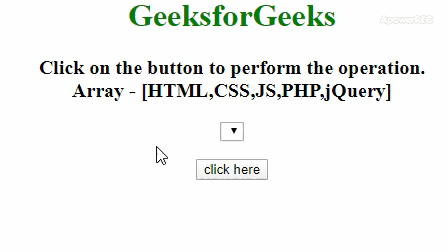Related Articles

# How to Create a Dropdown List with Array Values using JavaScript ?

• Last Updated : 30 Jul, 2021

The task is to add elements to the select element from the JavaScript array. We can also get selected value in dropdown list using JavaScript. Here we will populate the dropdown list with an array. Below is the description of popular approaches used in JavaScript.

Example 1: In this example, the length property is used to traverse the elements of the array and on each element create an option element and append this new element to the select element by appendChild() method

• Program:

## HTML

 ` ` `<``html``> ` ` `  `<``head``> ` `    ``<``title``> ` `        ``Populate dropdown list ` `        ``with array values ` `    `` ` ` ` ` `  `<``body` `style``=``"text-align:center;"``> ` `     `  `    ``<``h1` `style``=``"color:green;"``>  ` `        ``GeeksforGeeks  ` `    `` ` `     `  `    ``<``p` `id``=``"geeks"` `style``= ` `        ``"font-size:20px; font-weight:bold"``> ` `    `` ` ` `  `     `  `    ``<``select` `id``=``"arr"``> ` `     `  `    ``<``br``><``br``> ` `     `  `    ``<``button` `onclick``=``"GFG_Fun();"``> ` `        ``click here ` `    `` ` `     `  `    ``<``p` `id``=``"gfg"` `style="font-size: 26px; ` `        ``font-weight: bold;color: green;"> ` `    `` ` ` `  `     `  `    ``<``script``> ` `        ``var up = document.getElementById('geeks'); ` `        ``var down = document.getElementById('gfg'); ` `        ``var select = document.getElementById("arr"); ` `        ``var elmts = ["HTML", "CSS", "JS", "PHP", "jQuery"]; ` `        ``up.innerHTML = "Click on the button to " ` `                    ``+ "perform the operation"+ ` `                    ``".<``br``>Array - [" + elmts + "]"; ` ` `  `        ``// Main function ` `        ``function GFG_Fun() { ` `            ``for (var i = 0; i < ``elmts.length``; i++) { ` `                ``var ``optn` `= ``elmts``[i]; ` `                ``var ``el` `= ``document``.createElement("option"); ` `                ``el.textContent` `= ``optn``; ` `                ``el.value` `= ``optn``; ` `                ``select.appendChild(el); ` `            ``} ` `            ``down.innerHTML` `= ``"Elements Added"``; ` `        ``} ` `    `` ` ` ` ` `  ` `

• Output:Example 2: In this example, the each() method is used to traverse the elements of the array and on each element create an option element and append this new element to the select element by append() method in JQuery.

• Program:

## HTML

 ` ` `<``html``> ` ` `  `<``head``> ` `    ``<``title``> ` `        ``Populate dropdown list ` `        ``with array values ` `    `` ` `     `  `    ``<``script` `src``= ` `"https://ajax.googleapis.com/ajax/libs/jquery/3.4.1/jquery.min.js"``>  ` `    `` ` ` ` ` `  `<``body` `style``=``"text-align:center;"``> ` `     `  `    ``<``h1` `style``=``"color:green;"``>  ` `        ``GeeksforGeeks  ` `    `` ` `     `  `    ``<``p` `id``=``"geeks"` `style``= ` `        ``"font-size: 20px;font-weight: bold"``> ` `    `` ` ` `  `     `  `    ``<``select` `id``=``"arr"``> ` `     `  `    ``<``br``><``br``> ` `     `  `    ``<``button` `onclick``=``"GFG_Fun();"``> ` `        ``click here ` `    `` ` `     `  `    ``<``p` `id``=``"gfg"` `style="font-size: 26px; ` `        ``font-weight: bold; color: green;"> ` `    `` ` ` `  `     `  `    ``<``script``> ` `        ``var up = document.getElementById('geeks'); ` `        ``var down = document.getElementById('gfg'); ` `        ``var select = document.getElementById("arr"); ` `        ``var elmts = ["HTML", "CSS", "JS", "PHP", "jQuery"]; ` `        ``up.innerHTML = "Click on the button to " ` `                ``+ "perform the operation"+ ` `                ``".<``br``>Array - [" + elmts + "]"; ` ` `  `        ``// Main function ` `        ``function GFG_Fun() { ` `            ``\$.each(elmts, function(i, p) { ` `                ``\$('#arr').append(\$('<``option``>') ` `                            ``.val(p).html(p)); ` `            ``}); ` `            ``down.innerHTML = "Elements Added"; ` `        ``} ` `    `` ` ` ` ` `  ` `

• Output:CSS is the foundation of webpages, is used for webpage development by styling websites and web apps.You can learn CSS from the ground up by following this CSS Tutorial and CSS Examples.

Hey geek! The constant emerging technologies in the world of web development always keeps the excitement for this subject through the roof. But before you tackle the big projects, we suggest you start by learning the basics. Kickstart your web development journey by learning JS concepts with our JavaScript Course. Now at it’s lowest price ever!

My Personal Notes arrow_drop_up
Recommended Articles
Page :